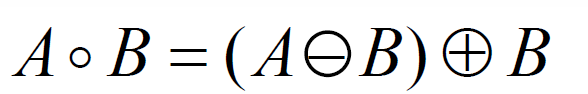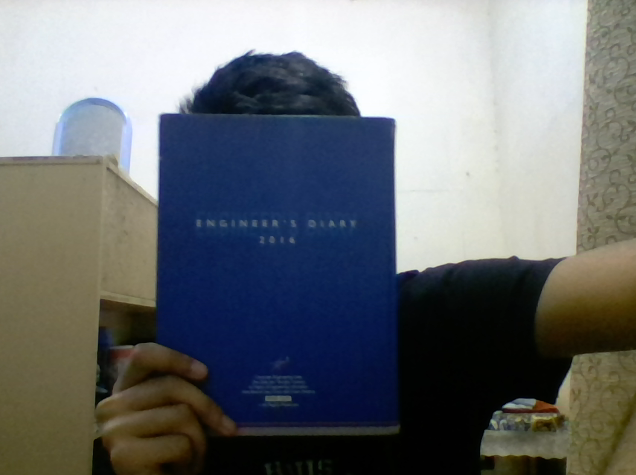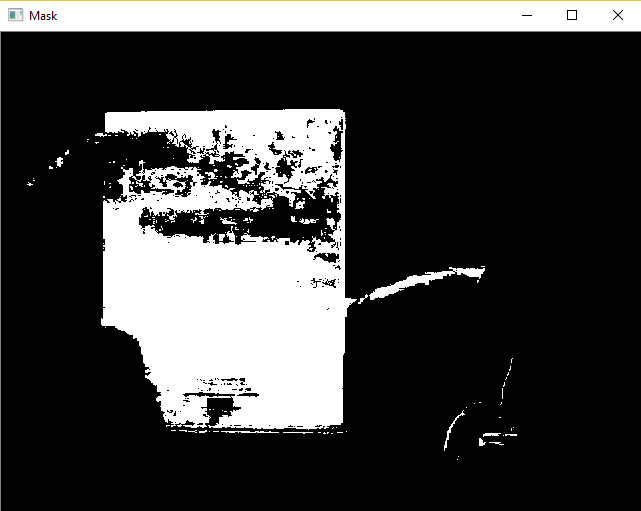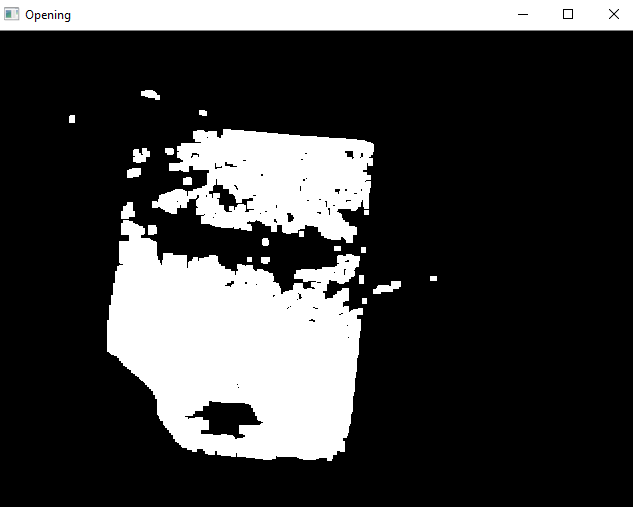Open In App
Related Articles

# Python | Morphological Operations in Image Processing (Opening) | Set-1

Morphological operations are used to extract image components that are useful in the representation and description of region shape. Morphological operations are some basic tasks dependent on the picture shape. It is typically performed on binary images. It needs two data sources, one is the input image, the second one is called structuring component. Morphological operators take an input image and a structuring component as input and these elements are then combines using the set operators. The objects in the input image are processed depending on attributes of the shape of the image, which are encoded in the structuring component.
Opening is similar to erosion as it tends to remove the bright foreground pixels from the edges of regions of foreground pixels. The impact of the operator is to safeguard foreground region that has similarity with the structuring component, or that can totally contain the structuring component while taking out every single other area of foreground pixels. Opening operation is used for removing internal noise in an image.
Opening is erosion operation followed by dilation operation.Syntax: cv2.morphologyEx(image, cv2.MORPH_OPEN, kernel)
Parameters:
-> image: Input Image array.
-> cv2.MORPH_OPEN: Applying the Morphological Opening operation.
-> kernel: Structuring element.

Below is the Python code explaining Opening Morphological Operation –

## Python3

 `# Python program to illustrate``# Opening morphological operation``# on an image`` ` `# organizing imports  ``import` `cv2  ``import` `numpy as np  `` ` `# return video from the first webcam on your computer.  ``screenRead ``=` `cv2.VideoCapture(``0``)`` ` `# loop runs if capturing has been initialized.``while``(``1``):``    ``# reads frames from a camera``    ``_, image ``=` `screenRead.read()``     ` `    ``# Converts to HSV color space, OCV reads colors as BGR ``    ``# frame is converted to hsv``    ``hsv ``=` `cv2.cvtColor(image, cv2.COLOR_BGR2HSV)``     ` `    ``# defining the range of masking``    ``blue1 ``=` `np.array([``110``, ``50``, ``50``])``    ``blue2 ``=` `np.array([``130``, ``255``, ``255``])``     ` `    ``# initializing the mask to be``    ``# convoluted over input image``    ``mask ``=` `cv2.inRange(hsv, blue1, blue2)`` ` `    ``# passing the bitwise_and over``    ``# each pixel convoluted``    ``res ``=` `cv2.bitwise_and(image, image, mask ``=` `mask)``     ` `    ``# defining the kernel i.e. Structuring element``    ``kernel ``=` `np.ones((``5``, ``5``), np.uint8)``     ` `    ``# defining the opening function ``    ``# over the image and structuring element``    ``opening ``=` `cv2.morphologyEx(mask, cv2.MORPH_OPEN, kernel)``    ` `    ``# The mask and opening operation``    ``# is shown in the window ``    ``cv2.imshow(``'Mask'``, mask)``    ``cv2.imshow(``'Opening'``, opening)``     ` `    ``# Wait for 'a' key to stop the program ``    ``if` `cv2.waitKey(``1``) & ``0xFF` `=``=` `ord``(``'a'``):``        ``break`` ` `# De-allocate any associated memory usage  ``cv2.destroyAllWindows()`` ` `# Close the window / Release webcam ``screenRead.release()`

Input Frame: